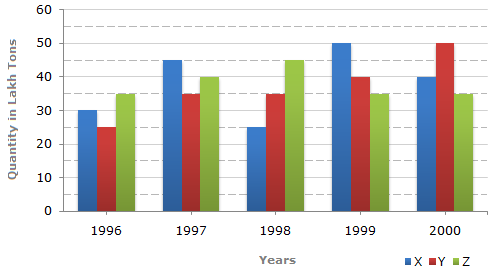# Data Interpretation - Bar Charts

Directions to Solve

The bar graph given below shows the data of the production of paper (in lakh tonnes) by three different companies X, Y and Z over the years.

Production of Paper (in lakh tonnes) by Three Companies X, Y and Z over the Years.1.
For which of the following years, the percentage rise/fall in production from the previous year is the maximum for Company Y?
1997
1998
1999
2000
Explanation:

Percentage change (rise/fall) in the production of Company Y in comparison to the previous year, for different years are:

 For 1997 =(35 - 25) x 100% = 40%. 25

 For 1998 =(35 - 35) x 100% = 0%. 35

 For 1999 =(40 - 35) x 100% = 14.29%. 35

 For 2000 =(50 - 40) x 100% = 25%. 40

Hence, the maximum percentage rise/fall in the production of Company Y is for 1997.

2.
What is the ratio of the average production of Company X in the period 1998-2000 to the average production of Company Y in the same period?
1:1
15:17
23:25
27:29
Explanation:

Average production of Company X in the period 1998-2000

 =1 x (25 + 50 + 40)=115lakh tons. 3 3

Average production of Company Y in the period 1998-2000

 =1 x (35 + 40 + 50)=125lakh tons. 3 3Required ratio =1153
= 115 = 23 .1253
125 25

3.
The average production for five years was maximum for which company?
X
Y
Z
X and Z both
Explanation:

Average production (in lakh tons) in five years for the three companies are:

 For Company X =1 x (30 + 45 + 25 + 50 + 40)= 190 = 38. 5 5

 For Company Y =1 x (25 + 35 + 35 + 40 + 50)= 185 = 37. 5 5

 For Company Z =1 x (35 + 40 + 45 + 35 + 35)= 190 = 38. 5 5Average production of five years is maximum for both the Companies X and Z.

4.
In which year was the percentage of production of Company Z to the production of Company Y the maximum?
1996
1997
1998
1999
Explanation:

The percentages of production of Company Z to the production of Company Z for various years are:

 For 1996 =35 x 100% = 140%. 25

 For 1997 =40 x 100% = 114.29%. 35

 For 1998 =45 x 100% = 128.57%. 35

 For 1999 =35 x 100% = 87.5%. 40

 For 2000 =35 x 100% = 70%. 50

Clearly, this percentage is highest for 1996.

5.
What is the percentage increase in the production of Company Y from 1996 to 1999?
30%
45%
50%
60%
 =(40 - 25) x 100% 25
 =15 x 100% 25